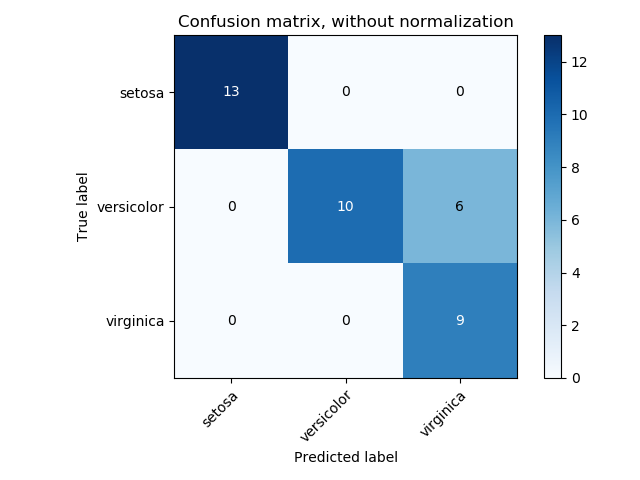## 一、分类

### 1. 精确率与召回率

$$\text{Precision}(A,B)=\frac{\|A\bigcap B\|}{\|A\|},\text{Recall}(A,B)=\frac{\|A\bigcap B\|}{\|B\|}$$。

$$F_{\beta}=(1+\beta^2)\cdot\frac{\text{precision}\cdot\text{recall}}{\beta^2\cdot\text{precision}+\text{recall}}$$。

### 2. ROC

ROC曲线适用于二分类问题，以假正率为横坐标，真正率为纵坐标的曲线图，如：AUC分数是曲线下的面积（Area under curve），越大意味着分类器效果越好。

### 3. 对数损失

$$L_\mathrm{ log}(y,p)=-\log\mathrm{ Pr}(y\|p)=-(y\log(p)+(1-y)\log(1-p))$$。

$$L_{\log}(Y_i,P_i)=-\log\mathrm{ Pr}(Y_i\|P_i)=\sum\limits_{k=1}^{K}y_{i,k}\log p_{i,k}$$。

### 4. 铰链损失

$$L_{\text{Hinge}}(w, y)=\max\{1-wy,0\}=\|1-wy\|_+$$。

$$L_{\text{Hinge}}(y_w, y_t)=\max\{1+y_t-y_w,0\}$$。

### 5. 混淆矩阵### 6. kappa系数

kappa系数（Cohen’s kappa）用来衡量两种标注结果的吻合程度，标注指的是把N个样本标注为C个互斥类别。计算公式为

$$\mathcal{K}=\frac{p_o-p_e}{1-p_e}=1-\frac{1-p_o}{1-p_e}$$。

GROUND
1 0
PREDICT 1 20 5
0 10 15

### 7. 准确率

$$\mathrm{ accuracy}=\frac{1}{n_\mathrm{ sample}}\sum\limits_{i=1}^{n_\mathrm{ sample}}1(\hat{y}_i=y_i)$$。

### 8. 海明距离

$$D_{Hamming}(\hat{y}_i,y_i)=\frac{1}{L}\sum\limits_{j=1}^L 1(\hat{y}_{ij}\neq y_{ij})$$。

### 9. 杰卡德相似系数

$$J(\hat{y}_i,y_i)=\frac{\|\hat{y}_i\bigcap y_i\|}{\|\hat{y_i}\bigcup y_i\|}$$。

### 10. 多标签排序

#### 10.1 涵盖误差

$$coverage(y,\hat{f})=\frac{1}{n_\text{samples}}\sum\limits_{i=1}^{n_\text{samples}}\max\limits_{j:y_{ij}=1}\text{rank}_{ij}$$，

#### 10.2 标签排序平均精度

$$LRAP(y,\hat{f})=\frac{1}{n_\text{samples}}\sum\limits_{i=1}^{n_\text{samples}}\frac{1}{\|y_i\|}\sum\limits_{j:y_{ij}=1}\frac{\|\mathcal{L}_{ij}\|}{\text{rank}_{ij}}$$，

#### 10.3 排序误差

$$ranking(y,\hat{f})=\frac{1}{n_\text{samples}}\sum\limits_{i=1}^{n_\text{samples}}\frac{1}{\|y_i\|(n_\text{labels}-\|y_i\|))}\left\|\mathcal{L}_{ij} \right\|$$，

## 二、回归

### 1. 平均绝对误差

$$\mathrm{ MAE}(y, \hat{y})=\frac{1}{n_\mathrm{ samples}}\sum\limits_{i=1}^{n_\mathrm{ samples}}\|y_i-\hat{y}_i\|$$。

### 2. 平均平方误差

$$\mathrm{ MSE}(y, \hat{y})=\frac{1}{n_\mathrm{ samples}}\sum\limits_{i=1}^{n_\mathrm{ samples}}(y_i-\hat{y}_i)^2$$。

### 3. 解释变异

$$\text{explained variance}(y,\hat{y})=1-\frac{\mathrm{Var} \{y-\hat y\}}{\mathrm{Var}\{y\}}$$。

#### 4. 决定系数

$$R^2(y,\hat{y})=1-\frac{\sum_{i=1}^{n_\mathrm{ samples}}(y_i-\hat{y}_i)^2}{\sum_{i=1}^{n_\mathrm{ samples}}(y_i-\bar{y})^2}$$，

## 三、聚类

### 1 . 兰德指数

$$\mathrm{RI}=\frac{a+b}{C_2^{n_\text{samples}}}$$，

$$\mathrm{ARI}=\frac{\mathrm{RI}-E[\mathrm{RI}]}{\max(\mathrm{RI})-E[\mathrm{RI}]}$$，

ARI取值范围为$$[-1,1]$$，值越大意味着聚类结果与真实情况越吻合。从广义的角度来讲，ARI衡量的是两个数据分布的吻合程度。

### 2. 互信息

$$H(U)=\sum\limits_{i=1}^{\|U\|}P(i)\log (P(i)), H(V)=\sum\limits_{j=1}^{\|V\|}P'(j)\log (P'(j))$$，

$$\mathrm{MI}(U,V)=\sum\limits_{i=1}^{\|U\|}\sum\limits_{j=1}^{\|V\|}P(i,j)\log\left ( \frac{P(i,j)}{P(i)P'(j)}\right )$$，

$$\mathrm{NMI}(U,V)=\frac{\mathrm{ MI}(U,V)}{\sqrt{H(U)H(V)}}$$。

$$\mathrm{ AMI}=\frac{\mathrm{ MI}-E[\mathrm{ MI}]}{\max(H(U), H(V))-E[\mathrm{ MI}]}$$。

### 3. 轮廓系数

$$s=\frac{b-a}{\max(a,b)}$$。